# Semi-continuous summation method

A summation method (cf. Summation methods) for series and sequences, defined by means of sequences of functions. Let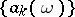,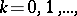be a sequence of functions defined on some setof variation of the parameter, and let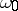be an accumulation point of(finite or infinite). The functions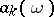are used to convert a given sequence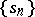into a function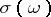:(1)

If the series in (1) is convergent for allsufficiently close to, and if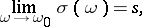one says that the sequenceis summable toby the semi-continuous summation method defined by the sequence. Ifis the sequence of partial sums of the series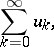(2)

one says that the series (2) is summable by the semi-continuous method to. A semi-continuous summation method withis an analogue of the matrix summation method defined by the matrix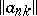, in which the integer-valued parameteris replaced by the continuous parameter. The sequence of functionsis therefore known as a semi-continuous matrix.

A semi-continuous summation method can be defined by direct transformation of a series into a function, using a given sequence of functions, say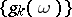: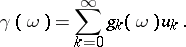(3)

In this case the series (2) is said to be summable toifwhereis an accumulation point of the setof variation of, and the series (3) is assumed to be convergent for allsufficiently close to.

In some cases a semi-continuous summation method is more convenient than a summation method based on ordinary matrices, since it enables one to utilize tools of function theory. Examples of semi-continuous summation methods are: the Abel summation method, the Borel summation method, the Lindelöf summation method, and the Mittag-Leffler summation method. The class of semi-continuous methods also includes methods with semi-continuous matrices of the form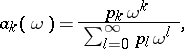where the denominator is an entire function that does not reduce to a polynomial.

Conditions for the regularity of semi-continuous summation methods are analogous to regularity conditions for matrix summation methods. For example, the conditionsfor allsufficiently close to,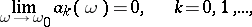are necessary and sufficient for the semi-continuous summation method defined by the transformation (1) ofinto a function to be regular (see Regularity criteria).